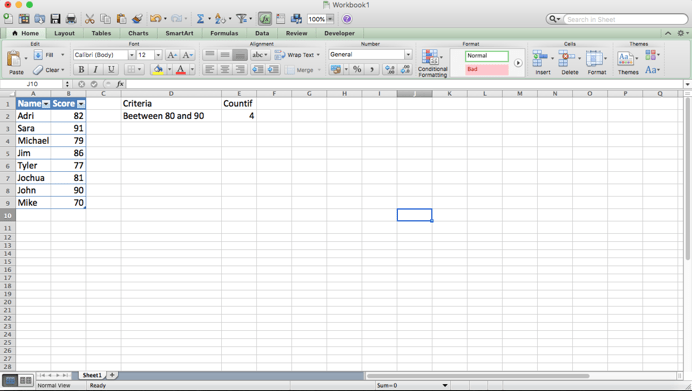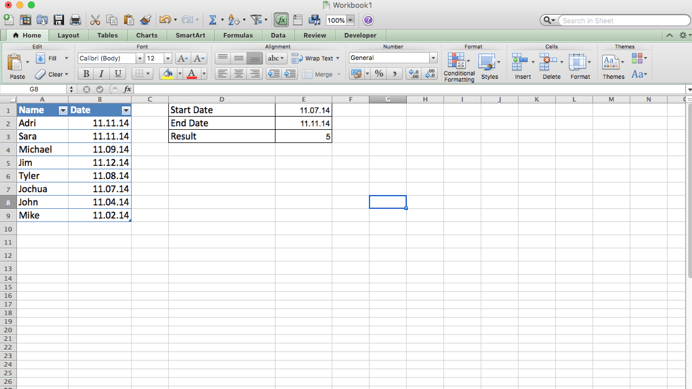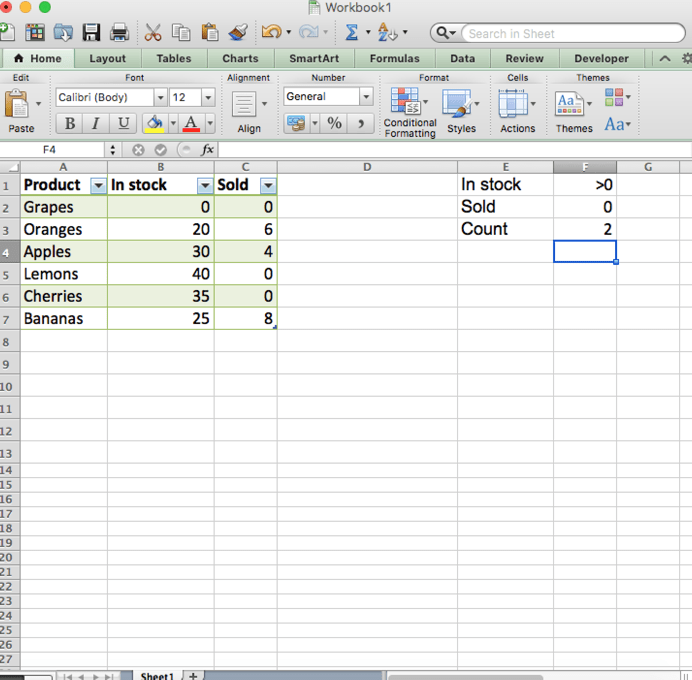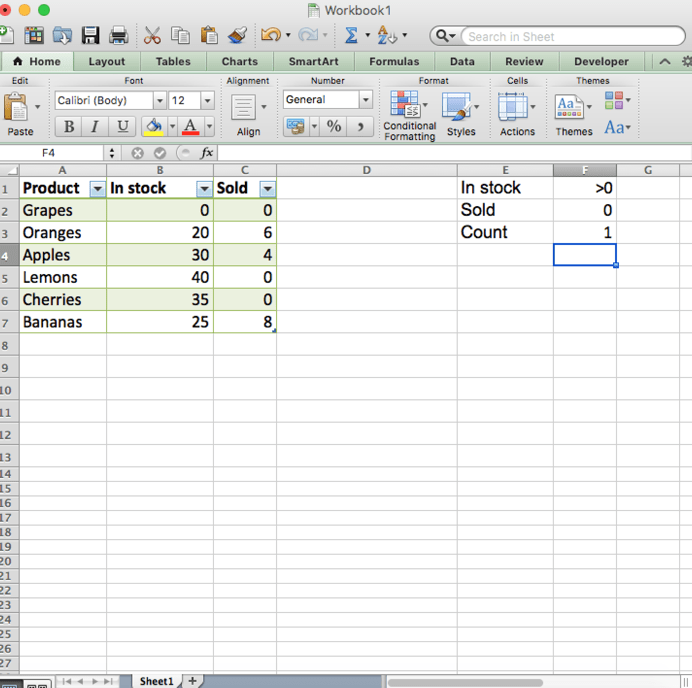Get instant live expert help with Excel or Google Sheets“My Excelchat expert helped me in less than 20 minutes, saving me what would have been 5 hours of work!”

#### Post your problem and you’ll get expert help in seconds.

Your message must be at least 40 characters
Our professional experts are available now. Your privacy is guaranteed.

# How to Use the COUNTIF Function to Count Cells Between Two NumbersRead time: 25 minutes

Using the COUNTIFS function you can achieve the result of counting numbers of cells which contain values between two numbers in a range.

## Using the COUNTIFS Function Between Two Values

The COUNTIFS function can show the count of cells that meet your criteria. You can use COUNTIF with criteria using dates, numbers, text and other conditions.

Using COUNTIFS you also need to use logical operators `(>,<,<>,=)`.

The COUNTIFS function is built to count cells that meet multiple criteria. In this case, because we supply the same range for two criteria, each cell in the range must meet both criteria in order to be counted.

Generic formula :

`=COUNTIFS(range,">=X",range,"<=Y")`Use `>=` for greater than or equal to

Use `<=` for less than or equal to

So if we want to count based on criteria : Between 80 and 90 in our table, we use this formula :

`=COUNTIFS(B2:B9,">=80",B2:B9,"<=90") `and the result should be 4 ( including : 82, 86, 81 and 90 )

### Using COUNTIFS between dates

The following COUNTIFS formula shows this idea by counting the number of dates that fall between a start and end date. The following formula counts the number of dates in A2:A9 that are equal to or greater than the date in E1 and equal to or less than the date in E2:

To count the number of cells that contain dates between two dates, you can use the COUNTIFS function:

`=COUNTIFS(range,">="&date1,range,"<="&date2)``=COUNTIFS(\$B\$2:\$B\$9,">="&\$E\$1,\$B\$2:\$B\$9,"<="&\$E\$2)`

So dates between 11/07/14 and 11/11/14 are found 5 dates, from B2 cell to B7 cell.

### The COUNTIFS formula with multiple criteria

Suppose you have a product list like in the example below, and you want to get a count of items that are in stock (value in column B is greater than 0) but have not been sold yet (value in column C is equal to 0).

The task can be accomplished by using this formula:

`=COUNTIFS(B2:B7,">0", C2:C7,"=0")`

And the count is 2 (“Cherries” and “Lemons”)When you want to count items with identical criteria, you still need to supply each criteria_range / criteria pair individually.For example, here’s the right formula to count items that have 0 both in column B and column C:

`=COUNTIFS(\$B\$2:\$B\$7,"=0", \$C\$2:\$C\$7,"=0")`

This COUNTIFS formula returns 1 because only “Grapes” have “0” value in both columns.

Different problems have their own best solution. If you are stuck and want to get to the solution quick, ask your question to have it answered by an Excel expert in 20 minutes. They are available to help you 24/7 at the link to the right. The first question is free.

### Did this post not answer your question? Get a solution from connecting with the expert.Another blog reader asked this question today on Excelchat:
Related blogs
Solution examplesI have a spreadsheet that contains a list of names/IDs and I want to generate a formula that inserts into a cell the number of times a name or ID appears on that list. Either insert a column into that spreadsheet or transfer that info into a column on a different spreadsheet. Either way would be fine.
Solved by O. L. in 18 minsI need a formula to count the number of rows that contain at least one of two possible phrases, but not give me the total number of instances that both phrases occur overall.
Solved by E. B. in 33 minsHello, I have a spreadsheet with 3159 rows. One column consists of 9-digit numbers in each of the 3159 rows. I need the formula that can calculate how many of those numbers appear more than once.
Solved by D. H. in 56 minsi need a formula to find values in column f in worksheet 1 and count them.
Solved by B. A. in 20 minsi have a table with Dates, First names , Last names and i want to count how many times a name occurs, but if a name occurs more than once on a particular date i need to only count it once. this is on excel and not google sheets
Solved by F. H. in 31 mins## Subscribe to Excelchat.coAnother blog reader asked this question today on Excelchat: